Next: Comparative Timing Results Up: User Testing Previous: Time Spent Entering and

## Parsing

When a formula is unparsable or the parser incorrectly interprets the formula, the user has to spend time rearranging the formula so that it is interpreted correctly. The third and fifth formulae, those which were integrals with limits, caused the most difficulty. Of the time users spent entering and correcting their formulae, 17% was spent making changes necessary to get the parser to interpret their formulae as desired.

As the parser was slow, a large proportion of the total time using the system, 41%, was spent by the user waiting for the parser to attempt to parse their formula. This time waiting for the parser also includes waiting for the system to generate a preview of their formula from the LATEX string generated. Preview generation typically takes 8 to 10 seconds per formula.

While the system was parsing the formulae, some participants took the opportunity to talk with the observer. Because they were talking, they did not notice the time that the system was taking to parse their formula and were less inclined to get impatient with the system. In one case a user waited two minutes before cancelling a parsing, but was talking at the same time. If they had not been talking, the user probably would probably have cancelled the formula processing sooner.

In the worst case, the amount of time taken to parse a formula is dependent on the size of the formula, the number of rules in the grammar, and the size of the individual rules within the grammar. The reason for this is that the search used by the system to match rules in the grammar with the current formula graph was a brute force approach that had very little optimisation. For example, when searching for a match of an integral rule, it is searching for a five-node subgraph within the main graph. The time taken is proportional to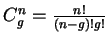where g is the number of nodes in the graph, and n is the number of nodes in the rule.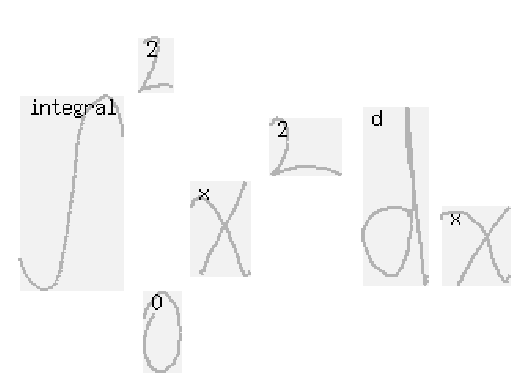(a) Input Formula.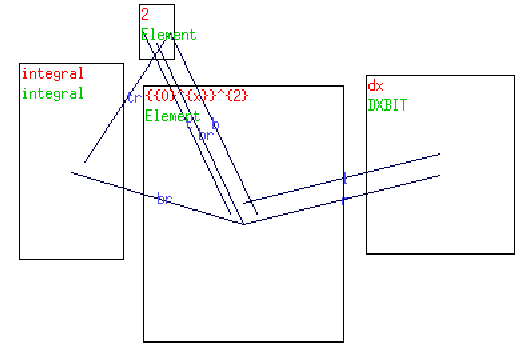(b) Misparsing in progress.

The most frustrating problem, from the user's point of view, is that the system spends a lot of time trying to complete a parsing after applying an incorrect rule early on. The system does eventually backtrack and correct itself, but can take several minutes to do so. Figure 6.8 illustrates this. The lower limit on the integral has been interpreted with the integrand as an exponent. Figure 6.8(a) is the formula entered by the user, and Figure 6.8(b) is the state of the graph part-way through the parsing process. The parser has initially taken the integral's limit 0 with the x2 as a power, giving 0x2.

The parser then collapses the integration term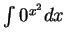, and finds the upper limit 2 is left over. The leftover symbol then causes the parser to backtrack and try an alternative interpretation of the formula.

While this is a non-catastrophic failure, it can take a very long time for the parser to eventually backtrack and correct this mistake, particularly if it is a large formula or this mistake happens early on in the parsing process.

Another problem was the accuracy of the parser, and its ability to interpret formulae correctly. For the formulae listed in Section 5.4, users took an average of one attempt to get formulae one to three to parse correctly. An average of four attempts were necessary for the more complex formulae four and five. Between parsing attempts, the user would make modifications to the positions of symbols in the formula.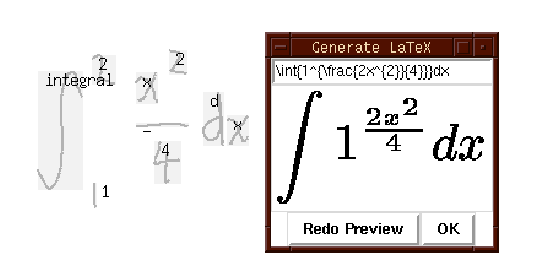At times formulae will parse, but the formula returned may not be the most plausible interpretation of a formula. The formula in Figure 6.9 shows an example of this. The integral's upper limit of 2 is multiplied by the x2, as it is approximately beside the x, and the entire fraction is raised as a power of the integral's lower limit of 1. The error is not detected by the parser as there are no left-over symbols.

The current, non-ideal, solution that the user must learn is to move the limits sufficiently far away from the integrand, so that the parser does not mistakenly incorporate them with the integrand.

Apart from the problem of the erroneous grouping of symbols, due to the lack of contextual tests and overly tolerant geometric layout tests, it handled the positional variations of handwritten input well. These problems only occurred when many symbols appeared close together, so if a user is aware they can avoid these problems with relative ease. In the user testing, it was eventually able to parse all the participants' handwritten formulae.

For the system to be of use to a mathematician, the range of formulae that the system accepts needs to be increased by adding to the underlying grammar. With the current implementation of the graph rewriting formula processor, this will increase processing times even more.Next: Comparative Timing Results Up: User Testing Previous: Time Spent Entering and
Steve Smithies
1999-11-13